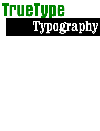TrueType Outlines

### Introduction

There are two key aspects that characterize the various outline font technologies: curve representation and hinting. TrueType hinting is discussed elsewhere. This page describes TrueType outlines.

### The Mathematics of TrueType Curves

TrueType's curves are quadratic B-splines. Each spline is equivalent to a series of quadratic Bézier curves. And each of these is defined by 3 outline control points, rendered by a parametric, quadratic equation hard-coded into the rasterizer.

If the 3 points of each Bézier curve are (Ax, Ay), (Bx, By) and (Cx, Cy), then

px = (1-t)2.Ax + 2t(1-t).Bx + t2.Cx
py = (1-t)2.Ay + 2t(1-t).By + t2.Cy

Varying the parameter t from 0 to 1 produces all the points p on the curve defined by A, B and C.

Visually, the curve runs between the two on-curve control points A and C, using the off-curve control point B to "pull" the curve in its direction. The curve is such that it leaves A along the straight line AB, and enters C along the straight line BC. (This property of the curves means that tangents are easy to define in TrueType.)The letter 'b' of Monotype Arial.
(TYPE*caster screen, with squares for on-curve points, crosses for off-curve points, blue lines for co-ordinate axes and bounding-box)
[Fig. 1]

In Figure 1, the points numbered 4, 5 and 6 correspond to the A, B and C of the above equations, and together define a section of the glyph outline. Where multiple off-curve points occur consecutively, an on-curve point is interpolated exactly half-way between them. Thus there is an implied on-curve point between points 7 and 8, between points 8 and 9, and so on. The curve running from point 6 to point 11 is a single quadratic B-spline, decomposed to 4 quadratic Bézier curves in the rasterizer.

Glyphs are defined as a series of contours, each of which contains three or more points. Each point is either on-curve or off-curve. Contours can even consist entirely of off-curve points.

Solid to the right
Solid shapes are defined by control points ordered clockwise. White space within solids are defined anti-clockwise. Thus, you can use the rule "solid to the right" as you traverse any part of an outline with point numbers increasing.

Badly-behaved outlines
Self-intersecting shapes (where clockwise and anti-clockwise lose their meanings) and overlapping shapes (where 'solidness' and 'whiteness' may conflict) are discouraged, because some output devices handle them poorly. However if you do choose to use them, you'll discover TrueType uses a non-zero winding fill algorithm.

### The Format of TrueType Outline Data

Outlines for all the glyphs in a font are stored in TrueType's 'glyf' table. The format is described with extreme conciseness, in just over 4 pages of the TrueType specification. This table, containing all the glyphs in order, is indexed by the 'loca' table.

There are three kinds of glyph in TrueType: simple, composite and zero-contour...

• Simple glyphs contain compressed outline data and hinting instructions.

• Composite glyphs refer to other glyphs for their outlines. Referenced components may be simple or composite themselves. A composite glyph inherits all the hinting in each of its components, and may be hinted further. The commonest use of composite glyphs is for the efficient representation of accented glyphs.
Each component in a composite glyphs has a 2×2 transformation matrix plus a translation. Unfortunately in Windows 3.1 this only worked for simple cases. Windows 95 and NT corrected the bug.

• Zero-contour glyphs, such as the space character, signify some behaviour in text but do not have a printed form. Zero-contour glyphs, like all glyphs, have a left side-bearing and advance width stored in the 'hmtx' table, but these metrics cannot be hinted as they can with simple and component glyphs.

### See also

TYPE*chimérique | TrueType Typography | TYPE*links